# The wettest June on record?

#### Part 1

Yesterday the BBC reported that the Met office records had June 2012 to be officially the “wettest June on record”.

In order to look at how the month really compared to previous years I downloaded the data and used R to analyse them quickly.

## Scraping data from the website

The metoffice data consists of text files for each region and each variable. Downloading all the data at once involves running a function multiple times that takes the file name as input.
In R the syntax “eval(parse(text=”“) evaluates any text as R code. If commands are pasted together either in a loop or by using lapply they can be run iteratively this way.

``````places <- c("England", "Scotland_N", "England_SW_and_S_Wales", "England_S",
"Scotland_W", "Scotland_E", "Scotland_W", "Midlands", "East_Anglia", "England_SE_and_Central_S",
"UK", "Wales", "England_N", "England_NW_and_N_Wales", "England_E_and_NE")

GetData <- function(what = "Rainfall", place = "England") {
what, "/date/", place, ".txt\",fill=T,skip=7,head=T)", sep = "")
eval(parse(text = a))
d <- data.frame(Region = place, Var = what, d)
}

Rain <- lapply(places, function(x) GetData(what = "Rainfall", place = x))
Rain <- do.call("rbind", Rain)
Tmean <- lapply(places, function(x) GetData(what = "Tmean", place = x))
Tmean <- do.call("rbind", Tmean)
Tmin <- lapply(places, function(x) GetData(what = "Tmin", place = x))
Tmin <- do.call("rbind", Tmin)
Tmax <- lapply(places, function(x) GetData(what = "Tmax", place = x))
Tmax <- do.call("rbind", Tmax)
Raindays <- lapply(places, function(x) GetData(what = "Raindays1mm",
place = x))
Raindays <- do.call("rbind", Raindays)
AirFrost <- lapply(places, function(x) GetData(what = "AirFrost",
place = x))
AirFrost <- do.call("rbind", AirFrost)
Sunshine <- lapply(places, function(x) GetData(what = "Sunshine",
place = x))
Sunshine <- do.call("rbind", Sunshine)

``````

It is worth saving this data locally in order to save time loading it again.

``````d <- rbind(Rain, Tmean, Tmax, Tmin, Raindays, AirFrost, Sunshine)
write.csv(d, file = "/home/duncan/Dropbox/Public/R/Data/UKClimate.csv",
row.names = F)
``````

## Plotting the time series of June rainfall

A good way to begin any analysis is to eyeball all the data at once. These are time series, so the best way is to plot out the variable as a line with the year on the x axis.
The ggplot2 library provides a poweful way of achieving this with a nice default style that looks more contemporary than lattice graphs.
The simplest way to use the package is to design a qplot. The "geom” arguments set the elements that are placed on the plot. The facets correspond to panels. By adding a loess smoother we can get an impression of the trend. The smoother has confidence intervals, so the significance of any trend can be evaluated by eye.

``````library(xtable)
library(ggplot2)
``````
``````## Need help? Try the ggplot2 mailing list:
``````
``````library(car)
``````
``````## Loading required package: nnet
``````
``````## Attaching package: 'car'
``````
``````## The following object(s) are masked from 'package:gtools':
##
## logit
``````
``````qplot(Year, JUN, colour = Region, facets = ~Region, geom = c("line",
"smooth"), data = Rain, method = loess, main = "June rainfall")
``````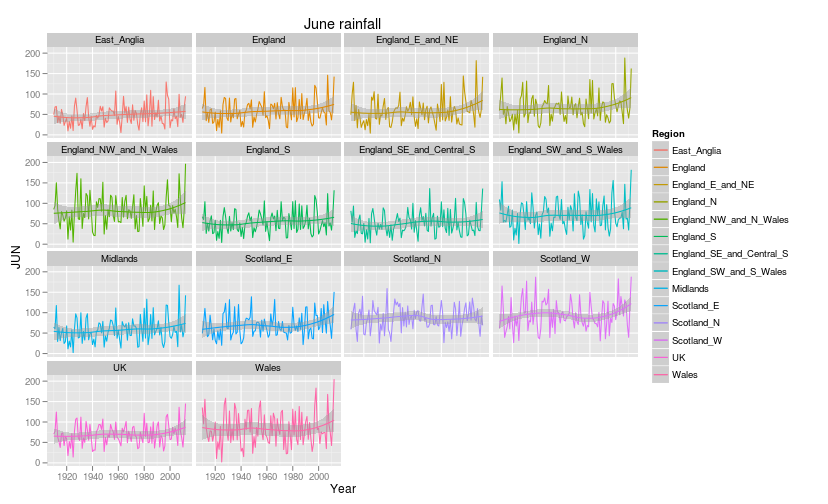## Looking at the distribution with boxplots

Inspection of the figures show that June 2012 was indeed the wettest on record for many regions and for the UK as a whole. Notice that 2007 was also nearly as wet in
some regions. A simple boxplot with 2012 marked as red points helps to show the pattern.

``````Rain\$Region <- reorder(Rain\$Region, Rain\$JUN, median)
par(mar = c(2, 8, 2, 2), cex.axis = 0.7)
R2012 <- subset(Rain, Year == 2012)
boxplot(JUN ~ Region, data = Rain, col = "grey", main = "June rainfall",
horizontal = T, las = 1, ylab = "")
points(as.numeric(Region) ~ JUN, pch = 21, bg = 2, data = R2012,
cex = 1.2)
grid()
``````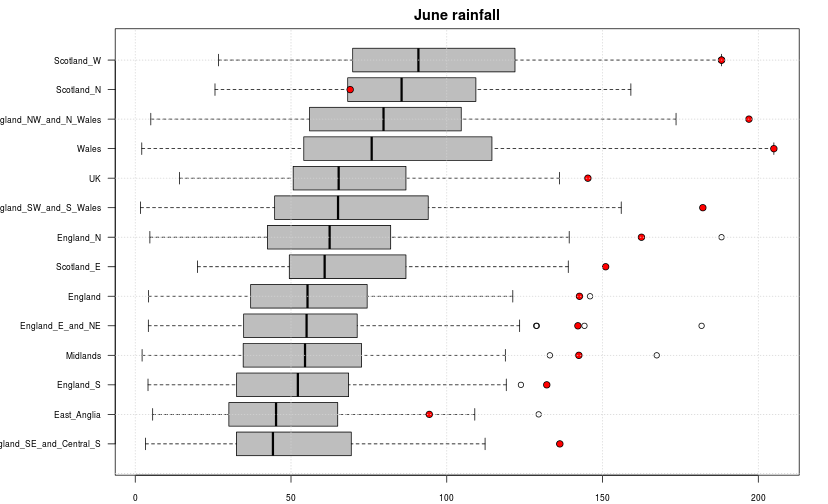So, in most regions the month stands out as a very distinct outlier. It also notable that the other outliers are from 2007. However June 2012 was not the wettest on record in the North of Scotland. East Anglia was not as unexpectedly wet as the rest of the UK either.

## Looking for a significant trend

If there is no consistent serial correlation between residuals and no evidence of a non linear trend, it may be justifiable to represent time series analysis as a simple linear regression. This has the advantage of simplicity and has a direct interpretation.

``````library(lmtest)
``````
``````## Loading required package: zoo
``````
``````## Attaching package: 'zoo'
``````
``````## The following object(s) are masked from 'package:base':
##
## as.Date, as.Date.numeric
``````
``````library(xtable)
par(mfrow = c(2, 2))
Rain2 <- split(Rain, Rain\$Region)
f <- function(x) {
x <- droplevels(x)
nm <- toupper(levels(x\$Region))
attach(x)
mod <- lm(JUN ~ Year, data = x)
an <- anova(mod)
pval <- round(an[1, ], 2)
mn <- round(mean(JUN), 2)
slp <- round(coef(mod), 2)
nm <- paste(nm, "\n", "P value=", pval)
nm <- paste(nm, "\n", "Mean=", mn, "mm")
nm <- paste(nm, "\n", "Change per decade=", slp * 10, "mm")
plot(JUN ~ Year, data = x, pch = 21, bg = 2, main = nm, type = "b", lty = 2,
cex.main = 0.95)
ablines(mod, lwd = 3)
grid()
detach(x)
dwtest(JUN ~ Year, data = x)
}
a <- lapply(Rain2, f)
``````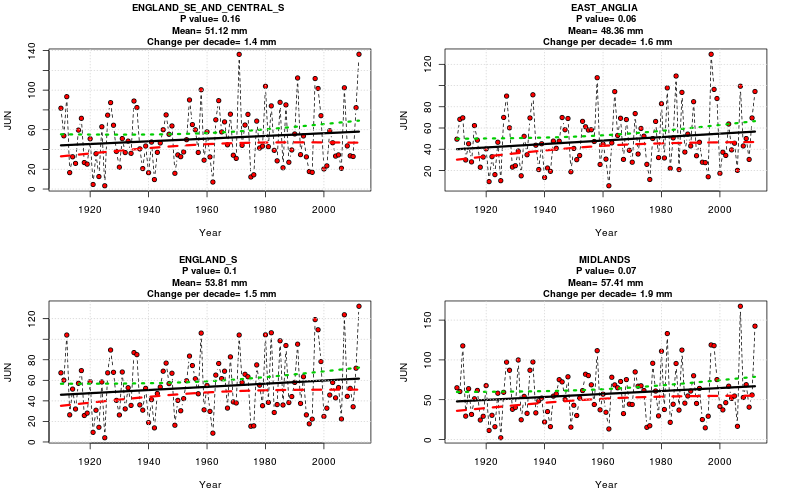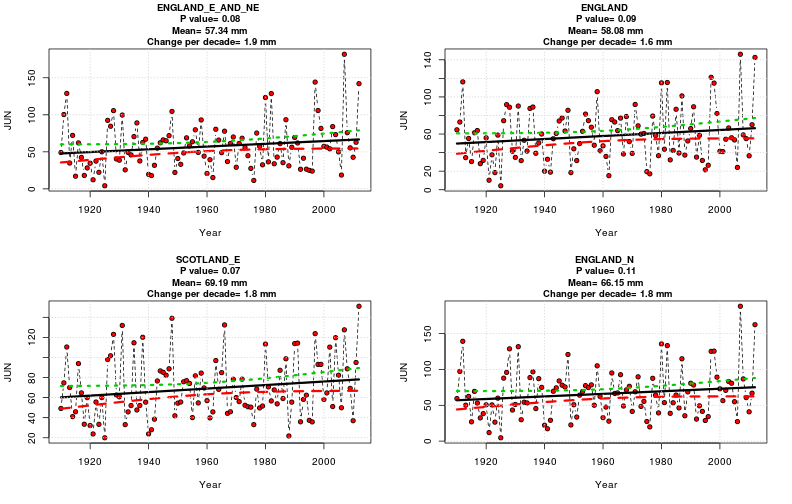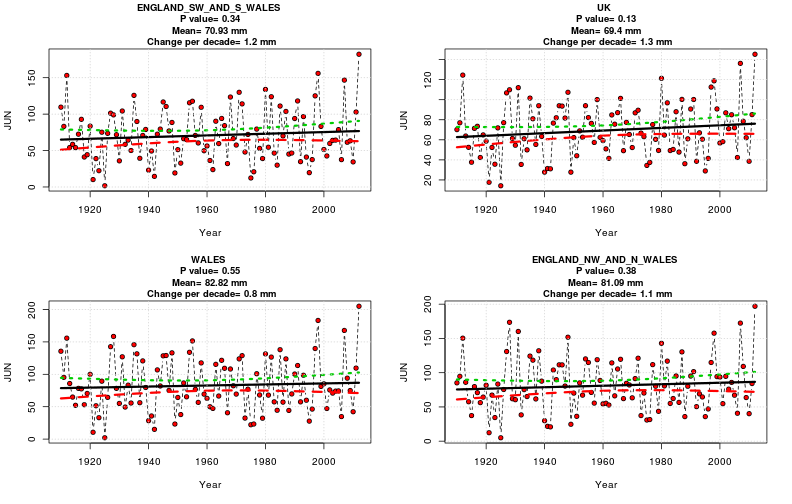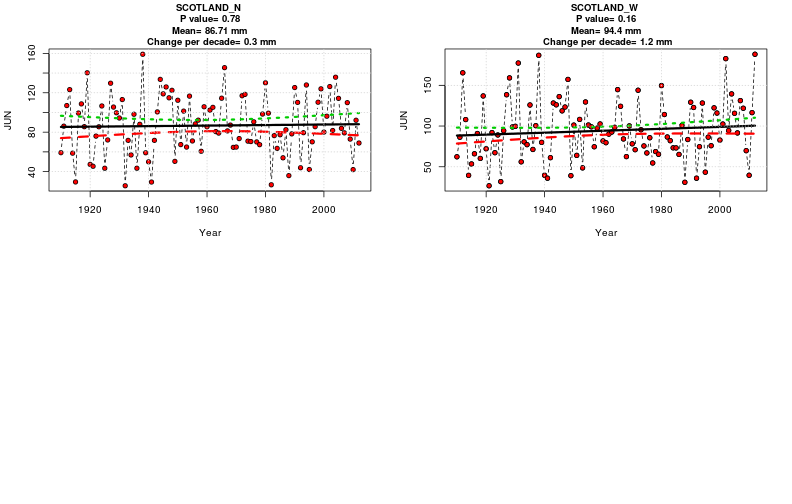``````dev.off()
``````

So, there is no evidence of statistically significant trends in any of the regions, although the trends are upwards. Even if there is a real increase in mean rainfall, the change in the mean value is small (<2mm per decade). So although June 2012 is exceptional, much more evidence is required in order to conclude that rainfall is consistently increasing over time.

The basis of the calculated p-values would be called into question by evidence of serial autocorrelation. The function returned the results of a Durbin-Watson test.

#### Are the residuals significantly autocorrelated?

``````print(xtable(data.frame(P_Value = unlist(lapply(a, function(x) x\$p.value)))),
type = "html")
``````
P_Value
England_SE_and_Central_S 0.33
East_Anglia 0.57
England_S 0.49
Midlands 0.79
England_E_and_NE 0.36
England 0.41
Scotland_E 0.21
England_N 0.31
England_SW_and_S_Wales 0.13
UK 0.16
Wales 0.14
England_NW_and_N_Wales 0.23
Scotland_N 0.15
Scotland_W 0.00

So, no evidence of serial autocorrelation. In other words last year's summer is not a very good predictor of this year's summer weather in a statistical sense. There are too few consistently long runs to form significant overall patterns. This is why meteorologists are better weather forecasters than climatologists.